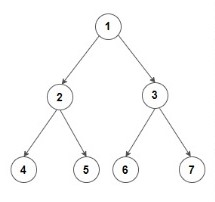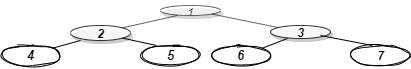New update is available. Click here to update.

# Bottom View Of Binary Tree

Last Updated: 24 Nov, 2020
Difficulty: Moderate

## PROBLEM STATEMENT

#### A node will be in the bottom-view if it is the bottom-most node at its horizontal distance from the root.

##### Note :
``````1. The horizontal distance of the root from itself is 0. The horizontal distance of the right child of the root node is 1 and the horizontal distance of the left child of the root node is -1.

2. The horizontal distance of node 'n' from root = horizontal distance of its parent from root + 1, if node 'n' is the right child of its parent.

3. The horizontal distance of node 'n' from root = horizontal distance of its parent from the root - 1, if node 'n' is the left child of its parent.

4. If more than one node is at the same horizontal distance and is the bottom-most node for that horizontal distance, including the one which is more towards the right.

5. You are not required to print the output explicitly, it has already been taken care of. Just implement the function and return the answer, which is the sequence of nodes from left to right in the bottom view.
``````

For example, for the given Binary Tree``````Below is the bottom view of the binary tree.
````````````1 is the root node, so its horizontal distance = 0.
Since 2 lies to the left of 0, its horizontal distance = 0-1= -1
3 lies to the right of 0, its horizontal distance = 0+1 = 1
Similarly, horizontal distance of 4 = Horizontal distance of 2 - 1= -1-1=-2
Horizontal distance of 5 = Horizontal distance of 2 + 1=  -1+1 = 0
Horizontal distance of 6 = 1-1 =0
Horizontal distance of 7 = 1+1 = 2

The bottom-most node at a horizontal distance of -2 is 4.
The bottom-most node at a horizontal distance of -1 is 2.
The bottom-most node at a horizontal distance of 0 is 5 and 6. However, 6 is more towards the right, so 6 is included.
The bottom-most node at a horizontal distance of 1 is 3.
The bottom-most node at a horizontal distance of 2 is 7.

Hence, the bottom view would be 4 2 6 3 7
``````
##### Input format :
``````The first line of input contains an integer ‘T’ denoting the number of test cases.
The next ‘T’ lines represent the ‘T’ test cases.

The first line of input contains the elements of the tree in the level order form separated by a single space.
If any node does not have a left or right child, take -1 in its place. Refer to the example below.
``````

Example :

``````Elements are in the level order form. The input consists of values of nodes separated by a single space in a single line. In case a node is null, we take -1 in its place.

For example, the input for the tree depicted in the below image would be :
```````````` 1
2 3
4 -1 5 6
-1 7 -1 -1 -1 -1
-1 -1

Explanation :
Level 1 :
The root node of the tree is 1

Level 2 :
Left child of 1 = 2
Right child of 1 = 3

Level 3 :
Left child of 2 = 4
Right child of 2 = null (-1)
Left child of 3 = 5
Right child of 3 = 6

Level 4 :
Left child of 4 = null (-1)
Right child of 4 = 7
Left child of 5 = null (-1)
Right child of 5 = null (-1)
Left child of 6 = null (-1)
Right child of 6 = null (-1)

Level 5 :
Left child of 7 = null (-1)
Right child of 7 = null (-1)

The first not-null node (of the previous level) is treated as the parent of the first two nodes of the current level. The second not-null node (of the previous level) is treated as the parent node for the next two nodes of the current level and so on.

The input ends when all nodes at the last level are null (-1).
``````
##### Note :
``````The above format was just to provide clarity on how the input is formed for a given tree.

The sequence will be put together in a single line separated by a single space. Hence, for the above-depicted tree, the input will be given as:

1 2 3 4 -1 5 6 -1 7 -1 -1 -1 -1 -1 -1
``````
##### Output format :
``````For each test case, the bottom view of the binary tree will be printed on a separate line with all the nodes included in the bottom view separated by a single space.
``````
##### Constraints :
``````1 <= T <= 100
1 <= N <= 3000
-10^9 <= DATA <= 10^9

Time Limit: 1 sec
``````## Approach 1

The idea is to take two arrays and to store horizontal distance in one and priority of each node in another. The arrays are of size ‘2*N+1’(considering the worst case). On traversing the tree, if the current calculated horizontal distance is not present in the array, add it. Otherwise, compare the priority(stored in the second array) of the previously-stored value with the current one. If the priority of the new node is greater, update the value in the array.

1. Take two vectors of size ‘2*N+1’ and initialize them to ‘INT_MAX’.
2. Initialize variable ‘MID’ as ‘N’ which is the position of the root node in the arrays. Also, initialize the variables ‘HORIZONTAL_DISTANCE’ and ‘PRIORITY’ to 0.
3. Take global variables ‘left’ and ‘right’, initialized to 0, denoting the size the nodes would take up in the arrays.
4. Make a function call to ‘BOTTOM_VIEW’.
5. In the function ‘BOTTOM_VIEW’,
a. If the root is NULL, return.
b. If the current horizontal distance is less than ‘LEFT’, update ‘LEFT’ to the value of horizontal distance.
c. If the current horizontal distance is greater than ‘RIGHT’, update ‘RIGHT’ to the value of horizontal distance.
d. If  ‘VEC[MID+HORIZONTAL_DISTANCE]’ is ‘INT_MIN’, that is, no node is present there, update ‘VEC[MID+HORIZONTAL_DISTANCE]’ to node’s data and ‘VEC[MID+HORIZONTAL_DISTANCE]’ to the current level number.
e. Else if a node’s value is already present at position  ‘VEC[MID+HORIZONTAL_DISTANCE]’, compare the priorities array of both the nodes and store the node with bigger priority at that position.
f. Recur for left subtree with ‘HORIZONTAL_DISTANCE-1’ and ‘PRIORITY+1’.
g. Recur for the right subtree with ‘HORIZONTAL_DISTANCE+1’ and ‘PRIORITY+1’.
6. Run a loop where ‘i’ ranges from ‘MID+LEFT’ to ‘MID+RIGHT’ and print all the values present in the first vector.